# Texas Go Math Grade 7 Lesson 10.3 Answer Key Lateral and Total Surface Area

Refer to our Texas Go Math Grade 7 Answer Key Pdf to score good marks in the exams. Test yourself by practicing the problems from Texas Go Math Grade 7 Lesson 10.3 Answer Key Lateral and Total Surface Area.

## Texas Go Math Grade 7 Lesson 10.3 Answer Key Lateral and Total Surface Area

Example 1
A net of a rectangular prism is shown. Use the net to find the lateral area and the total surface area of the prism. Each square represents one square inch. The blue regions are the bases, and the green regions are the lateral faces.Step 1
Find the lateral area of the rectangular prism. There are two 2 in. by 5 in. rectangles, and two 2 in. by 6 in. rectangles.

2 . (2 . 5) = 20 in2
2 . (2 . 6) = 24 in2
The lateral area is 20 + 24 = 44 in2.

Step 2
Find the total surface area of the rectangular prism.
Each base is 5 inches by 6 inches. 2 . (5 . 6) = 60 in2.
The total surface area is 44 + 60 = 104 in2.

Math Talk
Mathematical processes
Explain how the total surface area of a prism differs from the lateral area.

Question 1.
Use the net to find the lateral area and the total surface area of the triangular prism described by the net.Find the Lateral area of the triangular prism. We have:
One 4 cm. by 4 cm. rectangle;
One 4 cm. by 5 cm. rectangle;
One 3 cm. by 4 cm. rectangle.
1 . (4 . 4) = 16
1 . (4 . 5) = 20
1 . (3 . 4) = 12
The lateral area is 16 + 20 + 12 = 48 cm2.
Each base is 4 cm. by 3 cm.\
2 . (4 . 3) = 2 . 12 = 24 cm2
The total surfance area is 48 – 24 = 72 cm2.

Example 2
Use the net of this rectangular pyramid to find the lateral area and the total surface area of this pyramid.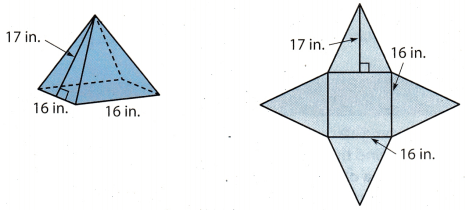Step 1
Find the lateral area of the rectangular pyramid. There are four triangles with base 16 in. and height 17 in. The lateral area is 4 × $$\frac{1}{2}$$(16)(17) = 544 in2.

Step 2
Find the total surface area of the rectangular pyramid.
The area of the base is 16 × 16 = 256 in2.
The total surface area is 544 + 256 = 800 in2.

Reflect

Question 2.
How many surfaces does a triangular pyramid have? What shape are they?
A triangular pyramid has four surfaces and they have the shape of triangle.

Question 3.
The base and all three faces of a triangular pyramid are equilateral triangles with side lengths of 3 ft. The height of each triangle is 2.6 ft. Use the net to find the lateral area and the total surface area of the triangular pyramid.Find the lateral area of the triangular pyramid.
We have three equilateral triangles with base 3 ft. and height 17 ft.
b = 3 ft Length of base
hB = 2.6 ft Height of base
Lateral area:
3 . ($$\frac{1}{2}$$ . b . hb) = 3 . $$\frac{1}{2}$$ . 3 . (2.6) = 11.7 ft2
The base is the same triangle as triangles in lateral faces of triangular pyramid
Therefore, area of the base is: $$\frac{1}{2}$$ . b . hb = 3.9 ft
The total surface area is 11.7 + 3.9 = 15.6 ft2.
The lateral area is 11.1 ft2.
The total surface area is 15.6 ft2.

Question 4.
Use a net to find the lateral area and the total surface area of the pyramid.Find the Lateral area of the rectangular pyramid.
We have four triangles with base 16 in. and height 20 in.
b = 16 ft Length of base
hB = 20 ft Height of base
Lateral area:
4. ($$\frac{1}{2}$$ . b. hb) = 4 . $$\frac{1}{2}$$ . 16 . 20 = 640 in2
The base is in the shape of rectangle.
Therefore, area of the base is: b . b = 256 in
The total surface area is 640 + 256 = 896 in2
The lateral area is 640 in2.
The total surface area is 896 in2.

Example 3.
Shoshanna’s team plans to build stands to display sculptures. Each stand will be in the shape of a rectangular prism. The prism will have a square base with side lengths of 2$$\frac{1}{2}$$ feet, and it will be 3$$\frac{1}{2}$$ feet high. The team plans to cover the stands with metallic foil that costs $0.22 per square foot. How much money will the team save on each stand if they cover only the lateral area instead of the total surface area? Step 1 Make a net of the rectangular prism.Step 2 Find the lateral and total surface areas of the prism. The four lateral faces are 3$$\frac{1}{2}$$ feet by 2$$\frac{1}{2}$$ feet rectangles. The two bases are 2$$\frac{1}{2}$$ feet by 2$$\frac{1}{2}$$ feet squares. Lateral area: 4$$\frac{1}{2}$$ . (3$$\frac{1}{2}$$ . 2$$\frac{1}{2}$$) = 35 square feet Total surface area: 2 (2$$\frac{1}{2}$$ . 2$$\frac{1}{2}$$) + 35 = 47$$\frac{1}{2}$$ square feet Add the area of the bases to the lateral area. Step 3 Find and compare the prices. Cost for total surface area:$0.22(47$$\frac{1}{2}$$) = $10.45 Cost for lateral area:$0.22(35) = $7.70 The team will save$10.45 – $7.70, or$2.75, for each stand by covering only the lateral area.

Question 5.
Kwame’s team will make two triangular pyramids to decorate the entrance to the exhibit. They will be wrapped in the same metallic foil. Each base is an equilateral triangle. If the base has an area of about 3.9 square feet, how much will the team save altogether by covering only the lateral area of the two pyramids?
The foil costs $0.22 per square foot. _______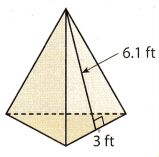Answer: Find the lateral area of the triangular pyramid We have three triangles with base of 3 ft and height of 6.1 ft. b = 3 ft Length of base hB = 6.1 ft Height of base Lateral area: 3. ($$\frac{1}{2}$$ . b. hb) = 3 . $$\frac{1}{2}$$ . 3. (6.1) = 27.45 ft2 Base area: B = 3.9 ft2 If they cover both whole pyramids they will spend: 2 (27.45 + 3.9) = 62.7 ft2< If we cover the lateral area of two pyramids we will spend: 2 . (27.45) = 54.9 ft2 The team will save: 0.22. (62.7 – 54.9) = 0.22. 7.8 =$1.7
The team will save $1.7 Texas Go Math Grade 7 Lesson 10.3 Guided Practice Answer Key A three-dimensional figure is shown sitting on a base. (Example 1) Question 1. The figure has a total of _____ rectangular faces. Answer: The figure has a total of six rectangular faces.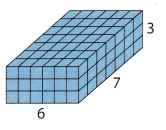Question 2. Of the total number of faces, ___ are lateral faces. Answer: Of the total number of faces, four are lateral faces Question 3. The figure is a ____. Answer: The figure is rectangular prism Question 4. Sketch a net of the figure. Answer: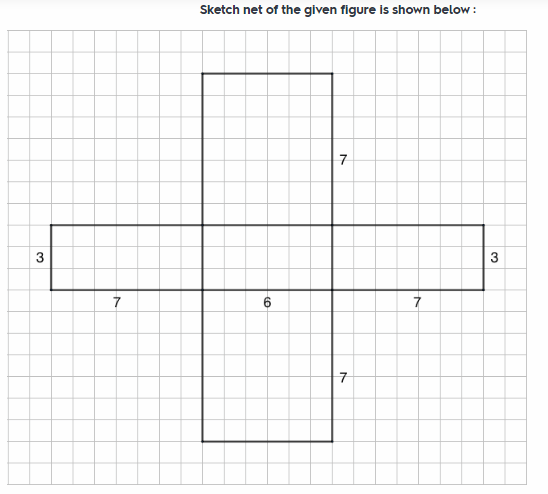Each box in the figure represent a single unit. Question 5. The lateral area of the prism is ___. Answer: For lateral area: Two 6 by 3 rectangtes Two 7 by 3 rectangles. 2 . (6 . 3) = 36 2 . (7 . 3) = 42 Lateral area: 36 + 42 = 78 The lateral area of the prism is 36 + 42 = 78 Question 6. The total surface area is ___. Answer: The base is in the shape of 6 by 7 rectangle. Base area: 2 (6 . 7) = 84 The total surface area is 78 + 84 = 162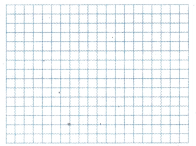A triangular prism is shown. (Example 2) Question 7. Identify the number and type of faces of the prism. Answer: A triangular prism has five face: Two 6 cm. by 4.3 cm. rectangles; One 6 cm. by 3 cm. rectangle; Two triangles with base of 3 cm. and height of 4 cm.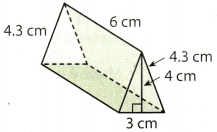Question 8. Find the lateral area of the prism. ____ Answer: For lateral area: Two 6 cm. by 4.3 cm. rectangles; One 6 cm. by 3 cm. rectangle; 2 . (6 . (4.3)) = 51.6 1 . (6 . 3) = 18 Lateral area: 51.6 + 18 = 69.6 cm2. The lateral area of the prism is 69.6 cm2. Question 9. Find the total surface area of the prism. Answer: The base is in the shape of triangle with length of 3 cm. and height of 4 cm. Base area 2 . ($$\frac{1}{2}$$ . 3 . 4) = 12 ft2 The total surface area is 69.6 + 12 = 81.6 cm2.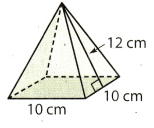Question 10. Use a net to find the total surface area of the pyramid. Then find the cost of wrapping the pyramid completely in gold foil that costs$0.05 per square centimeter. (Examples 2 and 3)
Find the lateral area of the rectangular pyramid.
we have four triangles with base of 10 cm. ain height of 12 cm.
b = 10 cm Length of base
hB = 12 cm Height of base
Lateral area:
4 . ($$\frac{1}{2}$$ . b . hb) = 4 . $$\frac{1}{2}$$ . 10 . 12 = 240 cm2
Base area:
10 . 10 = 1oo cm2
The total surface area is 240 + 100 = 340 cm2.
Wrapping the pyramid completely in gold foil will cost: 340 . (0.05) = \$17

Essential Question Check-In

Question 11.
How do you find the lateral and total surface area of a triangular pyramid?
Lateral surface area of any three-dimensional figure means the sum of area of all sides except the area of base. And the total surface area means the area of all the sides of figure including the area of base.

Now in case of triangular pyramid it will have total four faces including the base triangle. So the lateral surface area will. be the sum of areas of all the three triangle which are faces of triangular pyramid except the base triangle. And the total surface area will be the sum of areas of all the four triangle including the base triangle

Question 12.
Use a net to find the lateral area and the total surface area of the cereal box.
Lateral area: __________________________
Total surface area: _____________________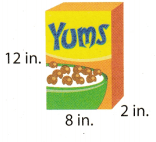The cereal box is in the shape of a rectangular prism.
Find the lateral area of the rectangular prism.
We have:
Two 2 in. by 12 in. rectangles;
Two 8 in. by 12 in. rectangles;
2 . (2 . 12) = 2 . 24 = 48
2 . (8 . 12) = 2 . 96 = 192
Lateral area: 48 – 192 = 240 in2.
Each base is 8 in. by 2 in.\
2 . (8 . 2) = 2 . 16 = 32 in2
The total surface area is 240 + 32 = 272 in2.
The lateral area is 240 in2.
The total surface area is 272 in2.

Question 13.
Describe a net for the shipping carton shown.
Net of the given rectangular prism or cuboid will consist of six rectangular surface with front and behind rectangle having dimension of 8 inch by 12 inch. And the dimension of top and bottom rectangle will be 8 inch by 2 inch.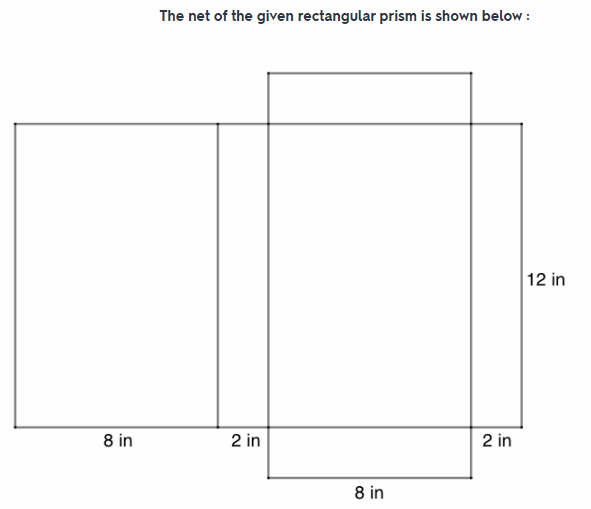Net of the given figure will consist of 6 rectangular surface.

Question 14.
A shipping carton is in the shape of a triangular prism.
Use a net to find the lateral area and the total surface area of the carton.
Lateral area: __________________________
Total surface area: _____________________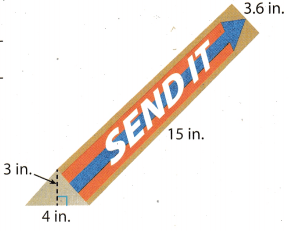Find the lateral area of the triangular prism.
We have:
Two 3.6 in. by 15 in. rectangles;
One 4 in. by 15 in. rectangles.
2. ((3.6) . 15) = 2 . 54 = 108
1 . (4 . 15) = 1 . 60 = 60
Lateral area: 108 + 60 = 168 in2
Each base has the shape of triangle with length of 8 in. and height of 12 in.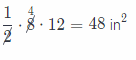Base Area
2 . 48 = 96 in2
The total surface area is 168 + 96 = 264 in2.

The lateral area of the carton is 168 in2.
The total surface area of the carton is 264 in2.

Question 15.
Victor wrapped this gift box with adhesive paper (with no overlaps). How much paper did he use?
The gift box is in the shape of a rectangular prism.
Find the lateral area of the rectangular prism.
We have:
Two 6 in. by 5 in. rectangle;
Two 8 in. by 5 in. rectangle.
2 . (6 . 5) = 60
2 . (8 . 5) = 80
The lateral area is 60 + 80 = 140 in2.
Each base is 8 in. by 6 in.\
2 . (8 . 6) = 2 . 48 = 96 in2
The total surfance area is 140 + 96 = 236 in2.

He used 236 in2 of paper.Question 16.
Vocabulary Name a three-dimensional shape that has four triangular faces and on rectangular face.
Three-dimensional. shape that has four triangular faces and one rectangular face is rectangular pyramid

Question 17.
Cindi wants to cover the top and sides of this box with glass tiles that are 1 cm square. How many tiles will she need?The box is in the shape of a rectangular prism.
Find the lateral area.
Two 15 cm. by 9 cm. rectangLe,
Two 20 cm. by 9 cm. rectangle;
2 . (15 . 9) = 270
2 . (20 . 9) = 360
Lateral area: 270 + 360 = 630 cm2.
The base is 20 cm. by 15 cm.
To cover a top of box we need a surface of one base.
1 . (20 . 15) = 1 . 300 = 300 cm2
To cover a top and sides of this box Cindi needs 630 + 300 = 930 cm2.
Therefore, she needs 930 tiles.
Cindi needs 930 tiles

Question 18.
A glass paperweight has the shape of a triangular prism. The bases are equilateral triangles with side lengths of 4 inches and heights of 3.5 inches. The height of the prism is 5 inches. Find the lateral area and the total surface area of the paperweight.
For lateral area:
There are three 4 in. by 5 in. rectangles.
3 . (4 . 5) = 3 . 20 = 60 in2
Lateral area: 60 in2
Base area:
The bases are equilateral triangles with length of 4 in. and height of 3.5 in.
2 . ($$\frac{1}{2}$$ . 4 . (3.5)) = 14 in2
The total surface area is 60 + 14 = 74 in2.

Lateral area: 60 in2.
The total surface area is 74 in2.

Question 19.
The doghouse shown has a floor, but no windows. Find the total surface area of the doghouse (including the door).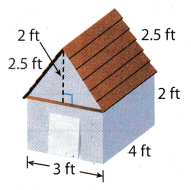Base of doghouse is in the shape of a rectangular prism.
For lateral area:
Two 4 ft. by 2 ft rectangles;
Two 3 ft by 2 ft. rectangles;
2 . (4 . 2) = 16
2 . (3 . 2) = 12
Lateral area: 16 + 12 = 28 ft2.
The base has the shape of rectangle 3 ft by 4 ft
Base area: 1 . (3 . 4) = 24 ft2
The total surface area is 28 + 24 = 52 ft2.
The roof of a doghouse is in the shape of triangular pyramid.
For lateral area:
Two 2.5 ft. by 4 ft. rectangles;
One 3 ft by 4 ft. rectangle.
2 . (2.5 . 4) = 20
1. (3 . 4) = 12
Lateral area: 20 + 12 = 32 ft2.
The base has the shape of triangle with length of 3 ft and height of 2 ft.
Base area:
2 . ($$\frac{1}{2}$$ . 3 . 2) = 6 ft2
The total surface area is 32 + 6 = 38 ft2.
The total surface area of doghouse is:
52 + 38 = 90 ft2

The total surface area of doghouse is 90 ft2.

Question 20.
Describe the simplest way to find the total surface area of a cube.
The surface area of a cube is the area of the six squares that covers it. The area of one of them is b. b. Since these are alt the same, multiply one of them by six, so the surface area of a cube is 6 times one of the sides squared.

Question 21.
Communicate Mathematical Ideas Describe how you approach a problem involving lateral area and total surface area. What do you do first? In what ways can you use the figure that is given with a problem? What are some shortcuts that you might use when you are calculating these areas?
When solving a problem involving lateral area and total surface area, determine first the lateral area of the given
figure. This is because the lateral area is needed to determine the total surface area of the given figure. It is easier to determine the lateral area of the given figure if you know what figure are you dealing with, is it a pyramid or a prism, and what is the base of the given figure. The total surface area is the sum of the lateral area and the area of the base. The lateral area should be determined first before determining the total surface area of the given figure.

Solve for the lateral area first.

Texas Go Math Grade 7 Lesson 10.3 H.O.T. Focus on Higher Order Thinking Answer Key

Question 22.
Persevere in Problem Solving A pedestal in a craft store is in the shape of a triangular prism. The bases are right triangles with side lengths of 12 cm, 16 cm, and 20 cm. The store owner used 192 cm2 of burlap cloth to cover the lateral area of the pedestal. Find the height of the pedestal.
Lateral area:
One 20 cm. by h cm. rectangle;
One 12 cm. by h cm. rectangle;
One 16 cm. by h cm. rectangle.
1 . (20 . h) = 20 . h.
1 . (12 . h) = 12 . h.
1 . (16 . h.) = 16 . h.
Lateral area: 20 . h. + 12 . h + 16 . h = 48 . h cm2.
The lateral area is 192 cm2.
192 = 48 . h.
Divide both sides by 48.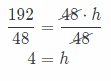The height of the pedestal is h = 4 cm.

Question 23.
Communicate Mathematical Ideas The base of Prism A has an area of 80 ft2, and the base of Prism B has an area of 80 ft2. The height of Prism A is the same as the height of Prism B. Is the base of Prism A congruent to the base of Prism B? Explain.
The base of Prism A has the same area with the base of Prism B. They could be congruent however, it depends on the shape of the base. If the bases of Prism A and Prism B are both squares, then they are congruent. But if the bases of Prism A and Prism B are triangle or rectangle, there could be a difference in the dimensions of the triangle base and the rectangle base, which makes them not congruent It is because congruent figures have the same shape and size.

It depends on the shape of the base.

Question 24.
Critique Reasoning A triangular pyramid is made of 4 equilateral triangles. The sides of the triangles measure 5 m, and the height of each triangle is 4.3 m. A rectangular prism has a height of 4.3 m and a square base that is 5 m on each side. Susan says that the total surface area of the prism is more than twice the total surface area of the pyramid. Is she correct? Explain.
Triangular pyramid:
For lateral area:
We have three triangles with base of 5 m. and height of 4.3 m.
b = 5m Length of base
hB = 4.3 m Height of base
Lateral area:
3. ($$\frac{1}{2}$$. b . hb) = 3 . $$\frac{1}{2}$$ . 5 . (4.3) = 32.25 m2
Lateral area: 32.25 m2.
The base is in the shape of triangle with base of 5 m. and height of 4.3 m
Base area: 1 . ($$\frac{1}{2}$$ . 5. (4.3)) = 10.75 m2
The total surface area is 32.25 + 10.75 = 43 m2
Rectangular prism:
For lateral area:
We have four 5 m. by 4.3 m. rectangles.
4 . (5 . (4.3)) = 86
Lateral area: 86 m2
The base is in the shape of 5 m. by 5 m. rectangle.
Base area:
2 (5 . 5) = 50 m2
The total surface area is 86 + 50 = 136 m2.Susan is correct. The total surface of area of the prism is three times of total surface area of the pyramid.

Scroll to Top